Question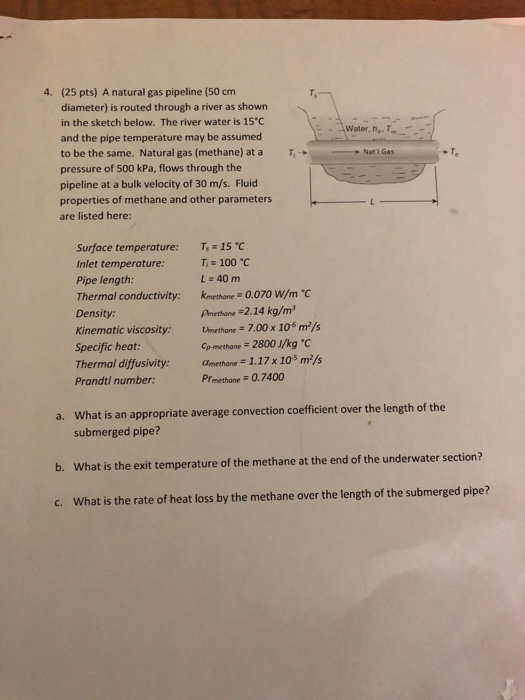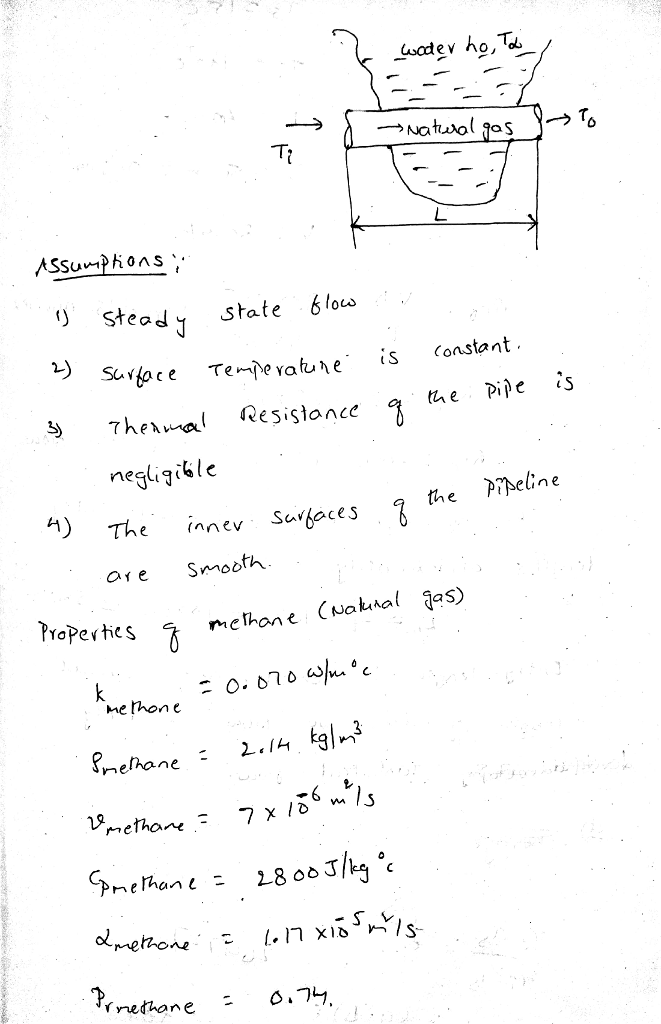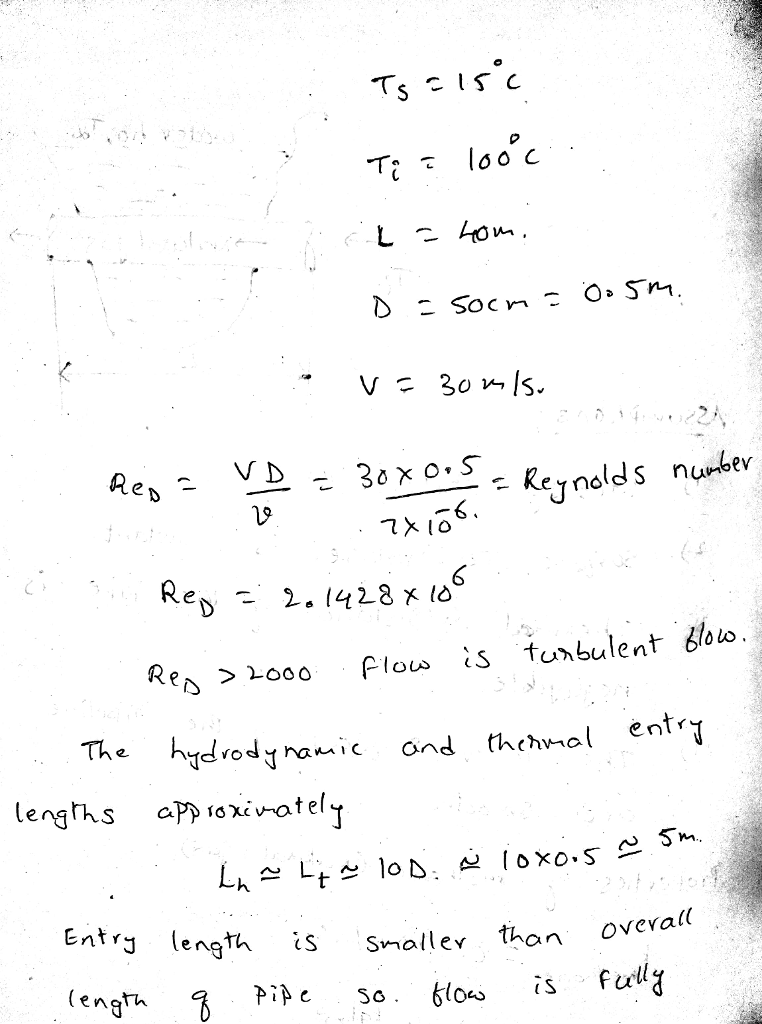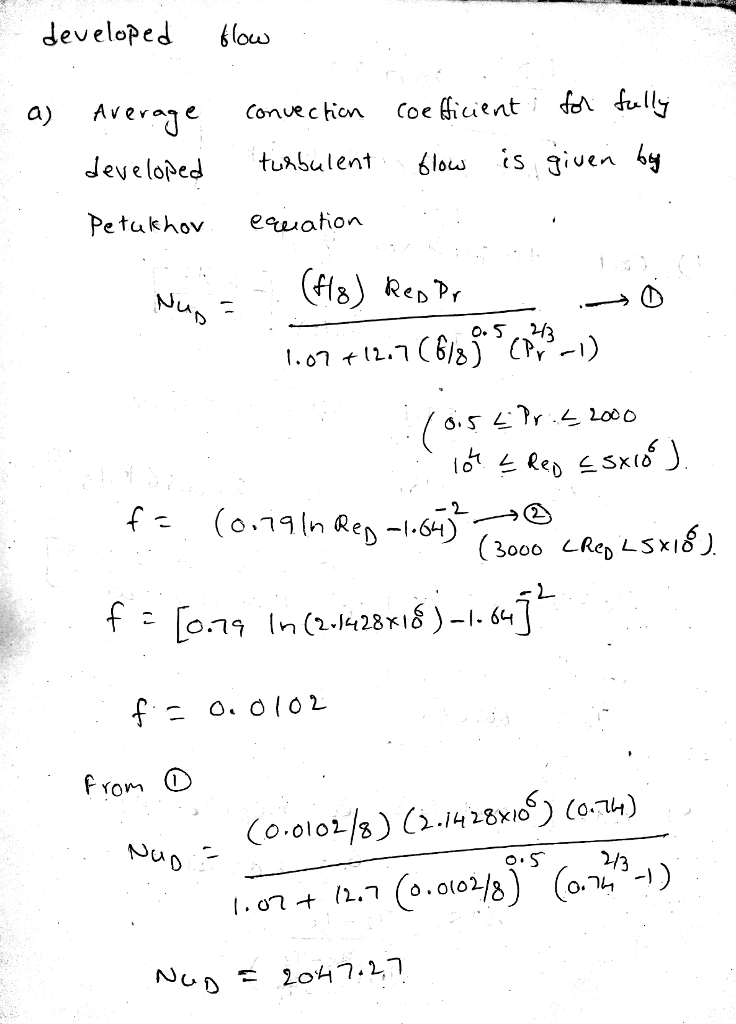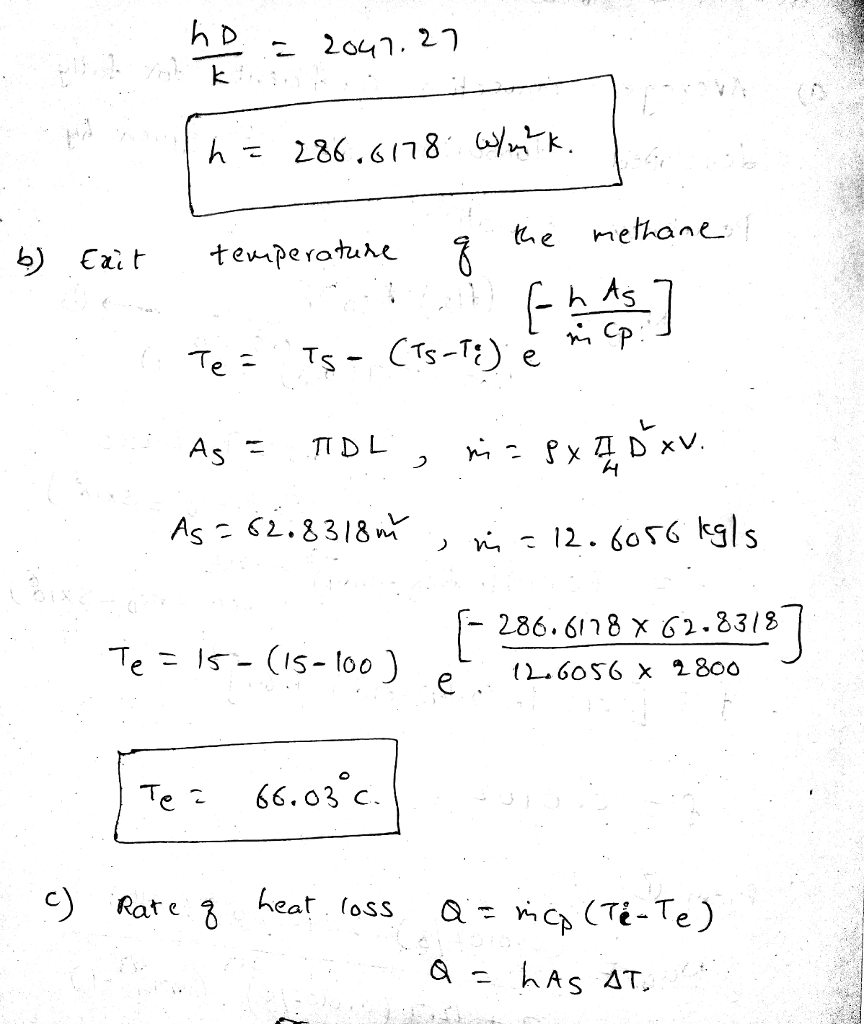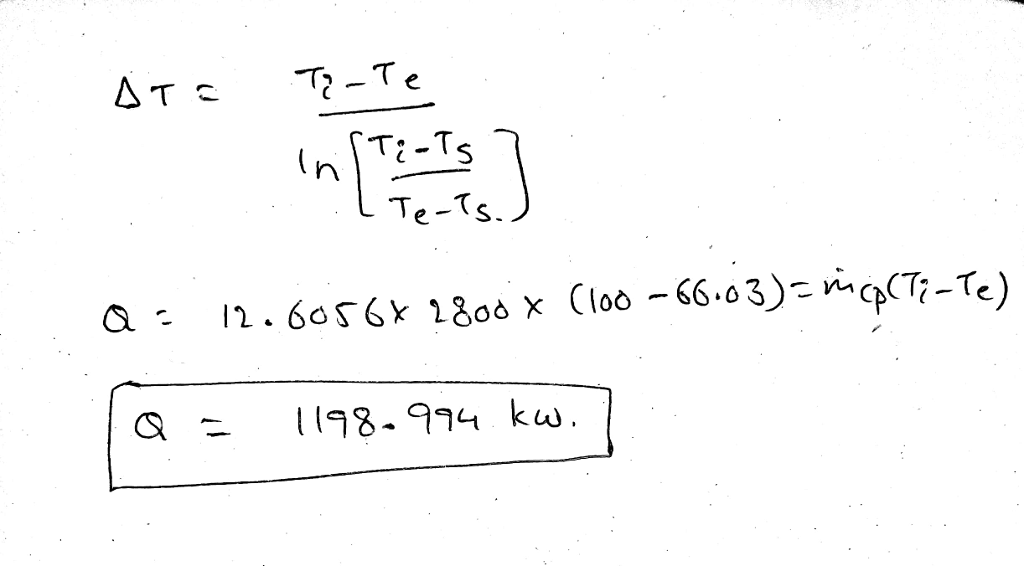#### Earn Coins

Coins can be redeemed for fabulous gifts.

Similar Homework Help Questions
• ### DON’T disregard the thermal conductivity of the pipe 3.) Oil at 200 C flows in a pipeline. The pipe has a 30-cm diameter and the flow is at an average velocity of 2 m/s. A 200-m-long section of t...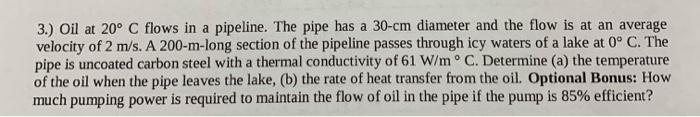DON’T disregard the thermal conductivity of the pipe 3.) Oil at 200 C flows in a pipeline. The pipe has a 30-cm diameter and the flow is at an average velocity of 2 m/s. A 200-m-long section of the pipeline passes through icy waters of a lake at 0° C. The pipe is uncoated carbon steel with a thermal conductivity of 61 W/m o C. Determine (a) the temperature of the oil when the pipe leaves the lake, (b) the...

• ### 4. (25 pts) Water is flowing inside a thin wall, smooth pipe of diameter 0.05 m...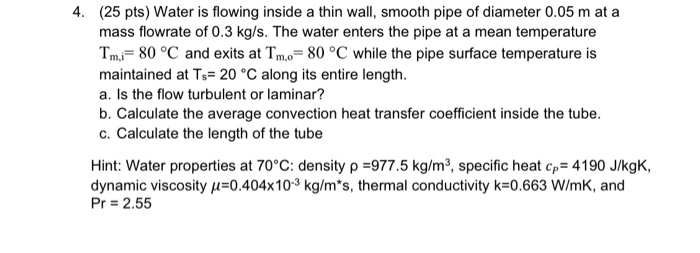4. (25 pts) Water is flowing inside a thin wall, smooth pipe of diameter 0.05 m at a mass flowrate of 0.3 kg/s. The water enters the pipe at a mean temperature Tm,i= 80 °C and exits at Tm,o= 80 °C while the pipe surface temperature is maintained at Ts= 20 °C along its entire length. a. Is the flow turbulent or laminar? b. Calculate the average convection heat transfer coefficient inside the tube. c. Calculate the length of the...

• ### Problem 3 (30): Steam at Too,1 340 °C flows in a cast iron pipe [k- 80 W/m.°C] whose inner and outer diameter are Di 6 cm and D2 -8 cm, respectively. The pipe is covered with a 4-cm thick glass wool...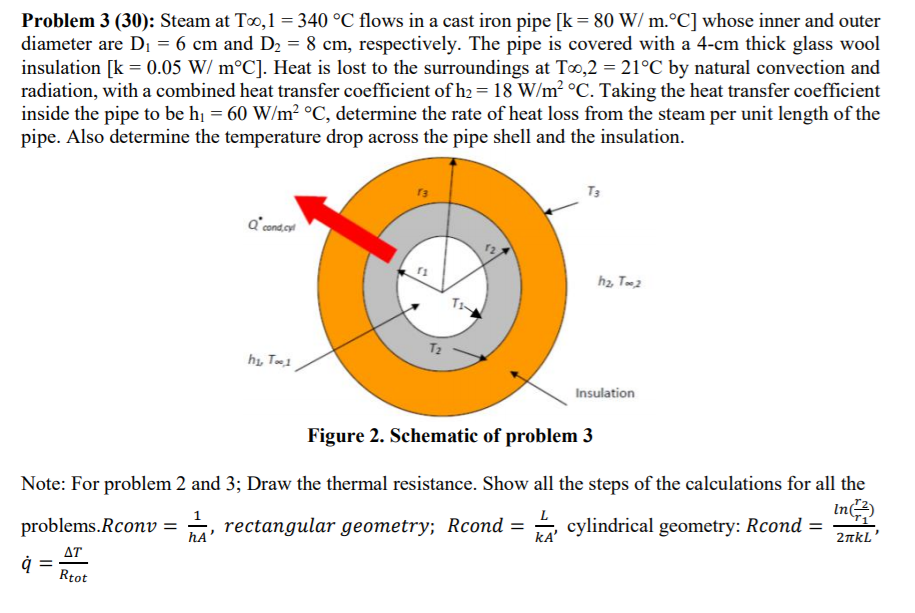Problem 3 (30): Steam at Too,1 340 °C flows in a cast iron pipe [k- 80 W/m.°C] whose inner and outer diameter are Di 6 cm and D2 -8 cm, respectively. The pipe is covered with a 4-cm thick glass wool insulation [k-0.05 W/ m°C]. Heat is lost to the surroundings at Too,2 - 21°C by natural convection and radiation, with a combined heat transfer coefficient of h- 18 W/m2 °C. Taking the heat transfer coefficient inside the pipe to...

• ### You are given a small pipe with a diameter of 1 mm flowing cooling fluid (water)...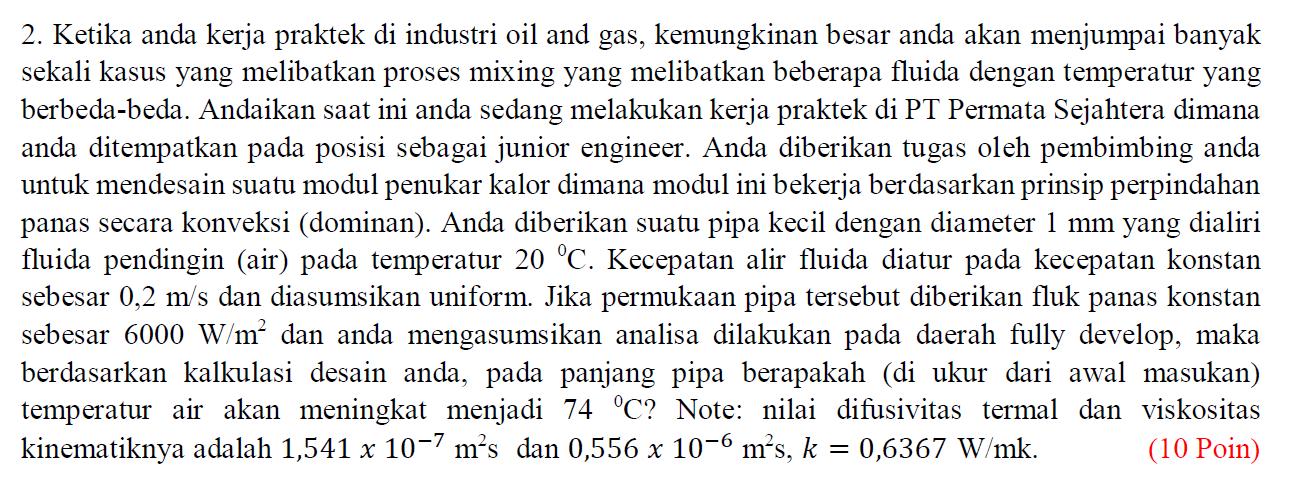You are given a small pipe with a diameter of 1 mm flowing cooling fluid (water) at a temperature of 20C. Fluid flow velocity is regulated at a constant speed of 0.2 m / s and assumed to be uniform. If the pipe surface is given a constant heat flux of 6000 W / m2 and you assume the analysis is done in a fully develop area, then based on your design calculations, at what length of the pipe (measured...

• ### 25 PRR HEAT TRANSFER NAME: UNIT SIX AND SEVEN EXCHANGERS BOILING AND CONDENSATION, He- Solve the following problems:...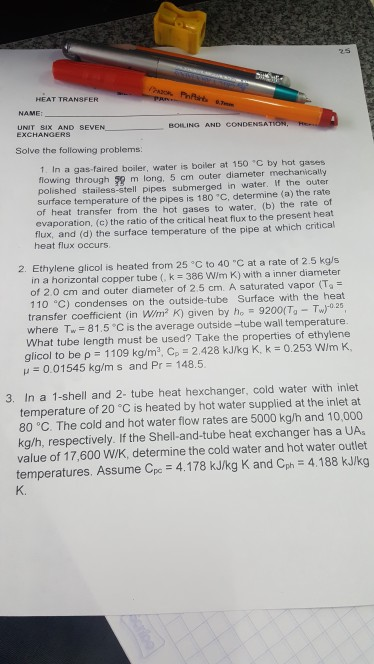25 PRR HEAT TRANSFER NAME: UNIT SIX AND SEVEN EXCHANGERS BOILING AND CONDENSATION, He- Solve the following problems: 1. In a gas-faired boiler, water is boiler at 150 C by hot gases flowing through 59 m long, 5 cm outer diameter mechanically polished stailess-stell pipes submerged in water, If the outer surface temperature of the pipes is 180 °C. determine (a) the rate of heat transfer from the hot gases to water. (b) the rate of evaporation, (c) the ratio...

• ### Waste heat of exhaust hot air from a manufacturing process may be recovered by passing water through a thin-walled tube of 1.00 cm diameter as shown. Assume the temperature of the hot air is 177℃ in...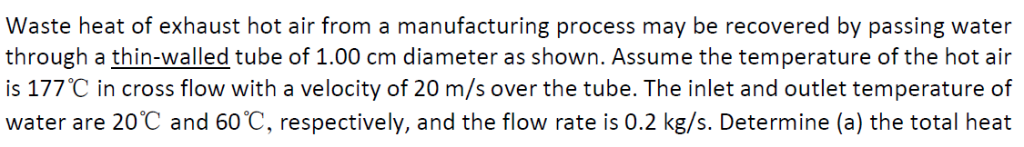Waste heat of exhaust hot air from a manufacturing process may be recovered by passing water through a thin-walled tube of 1.00 cm diameter as shown. Assume the temperature of the hot air is 177℃ in cross flow with a velocity of 20 m/s over the tube. The inlet and outlet temperature of water are 20C and 60 C, rospectively, and the ilow rate is 0.2 k/s. Deiemine (a) the total heat transfer rate from air to water, (b) The...